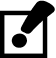# 3.3: Obtain dimensions from drawings

Obtain dimensions from drawings

The best way to get exact dimensions from drawings is to use the explicit dimensions (in millimeters or in feet and inches) written between the dimension lines. Any measurements that you need should be somewhere on the drawings. Drawings normally only give each dimension once. If there are a number of parallel lengths, only one will have a measurement. To find the dimension you need, you may need to refer to other views or you may have to add or subtract other dimensions.

Measuring lines on a drawing to determine the measurement is not an accurate way to extract dimensions. This is because the drawing is only a representation and may not be exact. Photocopies of drawings may not be to the scale of the original.

The scale of the drawing and your accuracy in measuring will lead to inaccuracies. For example, if the scale of a drawing is 1/8" = 1'-0", an error of 1/32" in measuring the plan amounts to 3" of error in the object measured. Detail drawings permit more exactness because they are proportionately larger. Details, however, often require more exactness and usually contain any needed dimensions.

When accuracy is not required and approximate dimensions are adequate, measuring plans is a quick method of taking off material for estimating the cost of a job. In such cases, 10% is usually added for cut-off and waste allowance.Measuring plans is not accurate enough for measuring materials for cutting and installation purposes.

If you use the scale of the drawing, it will be simple to read off the measurements. However, in the field you will often need approximate measurements and the only measuring tool at hand will be a measuring tape.

A steel pocket tape measure has a movable hook on the end that allows accurate measuring both when butted against a surface or when hooked on the end of an object (Figure 9). The end of the flexible tape itself is shortened to allow for the hook.1. Tape measure with movable hook

To avoid any error and in order to place the tape flat on the drawing, use a convenient unit mark, such as 100 mm, as the starting point for measuring, as in Figure 10.2. Measuring metric drawings

The distance between the dimension lines is 40 mm. Since the scale is 1:50, the centre to centre length is:

40 mm × 50 = 2000 mm

If the scale had been 1:100, the length would be 40 mm × 100 = 4000 mm.

Follow the same procedure to determine approximate lengths from imperial scale drawings. An imperial tape measure is used in the same way to find a length on the drawing in inches. As an example, suppose the length is 7½". To find the length represented, use the scale of the drawing.

In every case, if you divide the length on the drawing by the scale fraction or number, you will be calculating the length in feet. Since dividing by a fraction is the same as multiplying by its reciprocal, you multiply the drawn length in inches by:

4 when the scale is ¼" = 1'-0"

8 when the scale is 1/8" = 1'-0"

2 when the scale is ½" = 1'-0"The reciprocals of all standard scales used by architects are shown in Figure 11.

 Scale 3/32 1/8 3/16 1/4 3/8 1/2 3/4 1 1 1/2 (3/2) 3 Reciprocal 32/3 8 16/3 4 8/3 2 4/3 1 2/3 1/3
3. Reciprocals of standard scales

The 7½" length on a drawing scaled ¼" = 1'-0" would represent 7 ½" × 4 = 30'.

On a scale of 3/8" = 1'-0", the same 7 ½" drawing length would represent 7½" × 8/3 = 20'.4. Reading lengths of piping runs

Figure 12 is part of a piping run drawn to the scale of 1/8"= 1' -0". The total length of the run is 4¼" × w8 = 34".Now complete the Learning Task Self-Test.